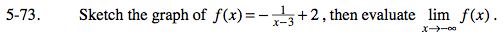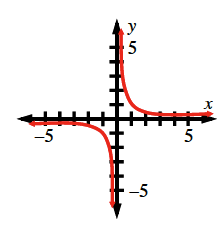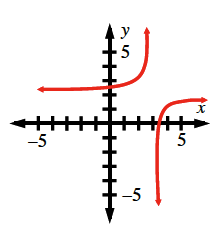### Home > PC > Chapter 5 > Lesson 5.2.3 > Problem5-73

5-73.$\text{The parent graph of } f(x) \text{ is } y = \frac{1}{x}.$

What does that graph look like?

$y=\frac{1}{x}$What does a negative sign do to a graph?
What about (x − 3) and + 2?Use the graph to evaluate the limit.